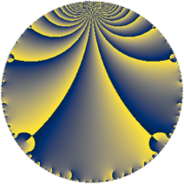# Properties

 Label 143.4.jLevel $143$ Weight $4$ Character orbit 143.j Rep. character $\chi_{143}(23,\cdot)$ Character field $\Q(\zeta_{6})$ Dimension $72$ Newform subspaces $1$ Sturm bound $56$ Trace bound $0$

# Related objects

## Defining parameters

 Level: $$N$$ $$=$$ $$143 = 11 \cdot 13$$ Weight: $$k$$ $$=$$ $$4$$ Character orbit: $$[\chi]$$ $$=$$ 143.j (of order $$6$$ and degree $$2$$) Character conductor: $$\operatorname{cond}(\chi)$$ $$=$$ $$13$$ Character field: $$\Q(\zeta_{6})$$ Newform subspaces: $$1$$ Sturm bound: $$56$$ Trace bound: $$0$$

## Dimensions

The following table gives the dimensions of various subspaces of $$M_{4}(143, [\chi])$$.

Total New Old
Modular forms 88 72 16
Cusp forms 80 72 8
Eisenstein series 8 0 8

## Trace form

 $$72 q - 12 q^{3} + 152 q^{4} + 90 q^{6} - 36 q^{7} - 360 q^{9} + O(q^{10})$$ $$72 q - 12 q^{3} + 152 q^{4} + 90 q^{6} - 36 q^{7} - 360 q^{9} - 56 q^{10} - 132 q^{12} - 46 q^{13} + 328 q^{14} - 644 q^{16} + 138 q^{17} + 492 q^{19} + 540 q^{20} - 44 q^{22} + 46 q^{23} + 720 q^{24} - 1636 q^{25} - 902 q^{26} + 48 q^{27} - 714 q^{28} - 262 q^{29} + 104 q^{30} + 144 q^{32} - 68 q^{35} + 1960 q^{36} + 630 q^{37} - 448 q^{38} - 1612 q^{39} + 216 q^{40} + 126 q^{41} - 82 q^{42} + 436 q^{43} - 570 q^{45} - 1590 q^{46} + 1944 q^{48} + 1192 q^{49} + 1290 q^{50} - 1424 q^{51} + 590 q^{52} + 292 q^{53} - 528 q^{54} - 440 q^{55} - 102 q^{56} - 1128 q^{58} + 504 q^{59} + 590 q^{61} + 1776 q^{62} + 1884 q^{63} - 5028 q^{64} + 994 q^{65} + 2156 q^{66} + 3396 q^{67} + 1530 q^{68} + 12 q^{69} - 1014 q^{71} - 1062 q^{72} - 1568 q^{74} + 4688 q^{75} + 7872 q^{76} - 1232 q^{77} - 4964 q^{78} - 2928 q^{79} - 558 q^{80} - 3780 q^{81} - 4072 q^{82} + 696 q^{84} + 4662 q^{85} + 1832 q^{87} + 924 q^{88} + 1014 q^{89} + 6844 q^{90} + 2900 q^{91} - 1336 q^{92} - 4092 q^{93} + 4166 q^{94} - 256 q^{95} - 5070 q^{97} - 8550 q^{98} + O(q^{100})$$

## Decomposition of $$S_{4}^{\mathrm{new}}(143, [\chi])$$ into newform subspaces

Label Dim $A$ Field CM Traces $q$-expansion
$a_{2}$ $a_{3}$ $a_{5}$ $a_{7}$
143.4.j.a $72$ $8.437$ None $$0$$ $$-12$$ $$0$$ $$-36$$

## Decomposition of $$S_{4}^{\mathrm{old}}(143, [\chi])$$ into lower level spaces

$$S_{4}^{\mathrm{old}}(143, [\chi]) \cong$$ $$S_{4}^{\mathrm{new}}(13, [\chi])$$$$^{\oplus 2}$$